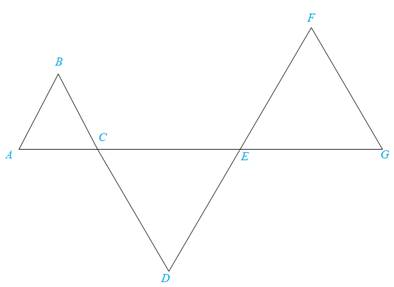Chapter 5.3, Problem 33EElementary Geometry For College St...

7th Edition
Alexander + 2 others
ISBN: 9781337614085

Solutions

Chapter
SectionElementary Geometry For College St...

7th Edition
Alexander + 2 others
ISBN: 9781337614085
Textbook Problem

In Exercise 33, provide a two-column proof.Given:                         A B ¯ ∥ D F ¯ , B D ¯ ∥ F G ¯ Prove:                         Δ A B C ∼ Δ E F GTo determine

To prove:

The statement ΔABCΔEFG if the sides AB¯ and DF¯ are parallel, AB¯DF¯ and the sides BD¯ and FG¯ are parallel, BD¯FG¯.

Explanation

Definition:

AA:

If two angles of one triangle are congruent to two angles of another triangle, then the triangles are similar.

Description:

Given that AB¯DF¯ and BD¯FG¯.

The given figure is shown below.

Figure

From the given figure, it is observed that the sides AB¯ and DF¯ are parallel, AB¯DF¯ and the sides BD¯ and FG¯ are parallel, BD¯FG¯.

Consider AB¯DF¯.

If two parallel lines are cut by a transversal, then the alternate interior angles are congruent.

Therefore, AFEG

Still sussing out bartleby?

Check out a sample textbook solution.

See a sample solution

The Solution to Your Study Problems

Bartleby provides explanations to thousands of textbook problems written by our experts, many with advanced degrees!

Get Started

In Exercises 19-24, find the functions f + g, f g, fg, and fg. 23. f(x) = x+1x1; g(x) = x+2x2

Applied Calculus for the Managerial, Life, and Social Sciences: A Brief Approach

Solve the equations in Exercises 126. 10x(x2+1)4(x3+1)510x2(x2+1)5(x3+1)4=0

Finite Mathematics and Applied Calculus (MindTap Course List)

Calculate y'. 45. y = ln( cosh 3x)

Single Variable Calculus: Early Transcendentals, Volume I

In Problems 15-20, find the indicated derivative. 15. .

Mathematical Applications for the Management, Life, and Social Sciences

True or False: is a convergent series.

Study Guide for Stewart's Multivariable Calculus, 8th

Think About It Explain why In ex= x.

Calculus: Early Transcendental Functions (MindTap Course List)